# Classifying Programming Languages

Classification is such an important exercise. It helps us to make sense of the world, and gives us a vocabulary. It helps us see patterns. And with thousands of incredibly diverse programming languages loose in the world, classifying them is a good use of our time.

## Overview

Different languages have different purposes, so it makes sense to talk about different kinds, or types, of languages. Some types are:

• Machine languages, that are interpreted directly in hardware
• Assembly languages, that are thin wrappers over a corresponding machine language
• High-level languages, that are anything machine-independent
• System languages, that are designed for writing low-level tasks, like memory and process management
• Scripting languages, that are generally extremely high-level and powerful
• Domain-specific languages, that are used in highly special-purpose areas only
• Visual languages, that are non-text based
• Esoteric languages, that are not really intended to be used, but are very interesting, funny, or educational in some way

These types are not mutually exclusive: Perl is both high-level and scripting; C is considered both high-level and system.

Other types people have identified: Toy, Educational, Very High-Level, Compiled, Interpreted, Free-Form, Curly Brace, Applicative, Von Neumann, Expression-Oriented, Persistent, Concurrent, Glue, Intermediate, Quantum, Hybrid. See Wikipedia’s category page on programming language classification.

## Machine Code

Most computers work by executing stored programs in a fetch-execute cycle. Machine code generally features:

• Registers to store values and intermediate results
• Very low-level machine instructions (`add`, `sub`, `div`, `sqrt`)
• Labels and conditional jumps to express control flow
• A lack of memory management support — programmers do that themselves

Machine code is usually written in hex. Here’s an example for the Intel 64 architecture:

```89 F8 A9 01 00 00 00 75 06 6B C0 03 FF C0 C3 C1 E0 02 83 E8 03 C3
```

Can you tell what it does?

## Assembly Language

An assembly language is an encoding of machine code into something more readable. It assigns human-readable labels (or names) to storage locations, jump targets, and subroutine starting addresses, but doesn’t really go too far beyond that. Here’s the function from above on the Intel 64 architecture using the GAS assembly language:

```        .globl  f
.text
f:
mov     %edi, %eax      # Put first parameter into eax register
test    \$1, %eax        # Examine least significant bit
imul    \$3, %eax        # It's even, so multiply it by 3
inc     %eax            # and add 1
ret                     # and return it
odd:
shl    \$2, %eax         # It's odd, so multiply by 4
sub    \$3, %eax         # and subtract 3
ret                     # and return it```

And here’s the same function, written for the SPARC:

```        .global f
f:
andcc   %o0, 1, %g0
bne     .L1
sll     %o0, 2, %g2
sll     %o0, 1, %g2
b       .L2
.L1:
.L2:
retl
nop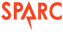```

## High-Level Languages

A high-level language gets away from all the constraints of a particular machine. HLLs have features such as:

• Names for almost everything: variables, types, subroutines, constants, modules
• Complex expressions (e.g. `2 * (y^5) >= 88 && sqrt(4.8) / 2 % 3 == 9`)
• Control structures (conditionals, switches, loops)
• Composite types (arrays, structs)
• Type declarations
• Type checking
• Easy, often implicit, ways to manage global, local and heap storage
• Subroutines with their own private scope
• Abstract data types, modules, packages, classes
• Exceptions

The previous example looks like this in Fortran 77 (note how the code begins in column 7 or beyond):

```       INTEGER FUNCTION F(N)
INTEGER N
IF (MOD(N, 2) .EQ. 0) THEN
F = 3 * N + 1
ELSE
F = 4 * N - 3
END IF
RETURN
END```

and like this in Fortran 90 (where the column requirements were finally removed):

```integer function f (n)
implicit none
integer, intent(in) :: n
if (mod(n, 2) == 0) then
f = 3 * n + 1
else
f = 4 * n - 3
end if
end function f```

```function F (N: Integer) return Integer is
begin
if N mod 2 = 0 then
return 3 * N + 1;
else
return 4 * N - 3;
end if;
end F;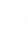```

and like this in C and C++:

```int f(const int n) {
return (n % 2 == 0) ? 3 * n + 1 : 4 * n - 3;
}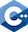```

and like this in Java and C#:

```class ThingThatHoldsTheFunctionUsedInTheExampleOnThisPage {
public static int f(int n) {
return (n % 2 == 0) ? 3 * n + 1 : 4 * n - 3;
}
}```

and like this in Scala:

```def f(n: Int) = if (n % 2 == 0) 3 * n + 1 else 4 * n - 3;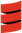```

and like this in Kotlin:

```fun f(n: Int) = if (n % 2 == 0) 3 * n + 1 else 4 * n - 3```

and like this in JavaScript:

```function f(n) {
return (n % 2 === 0) ? 3 * n + 1 : 4 * n - 3;
}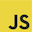```

and like this in CoffeeScript:

```f = (n) -> if n % 2 == 0 then 3 * n - 1 else 4 * n + 3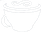```

and like this in Smalltalk:

```f
^self % 2 = 0 ifTrue:[3 * self + 1] ifFalse:[4 * self - 3]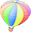```

and like this in Standard ML:

```fun f n = if n mod 2 = 0 then 3 * n + 1 else 4 * n - 3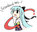```

and like this in Elm:

```f n = if n % 2 == 0 then 3 * n + 1 else 4 * n - 3```

and like this in Haskell (thanks @kaftoot):

```f n | even(n) = 3 * n + 1  | otherwise = 4 * n - 3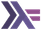```

and like this in Julia (yes, 3n is “three times n”):

```f(n) = iseven(n) ? 3n+1 : 4n-3```

and like this in Lisp:

```(defun f (n)
(if (= (mod n 2) 0)
(+ (* 3 n) 1)
(- (* 4 n) 3)))```

and like this in Clojure:

```(defn f [n]
(if (= (mod n 2) 0)
(+ (* 3 n) 1)
(- (* 4 n) 3)))```

and like this in Prolog:

```f(N, X) :- 0 is mod(N, 2), X is 3 * N + 1.
f(N, X) :- 1 is mod(N, 2), X is 4 * N - 3.
```

and like this in Erlang:

```f(N) when (N band 1) == 0 -> 3 * N + 1;
f(N) -> 4 * N - 3.```

and like this in Perl:

```sub f {
my \$n = shift;
\$n % 2 == 0 ? 3 * \$n + 1 : 4 * \$n - 3;
}```

and like this in Python:

```def f(n):
return 3 * n + 1 if n % 2 == 0 else 4 * n - 3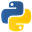```

and like this in Ruby:

```def f(n)
n % 2 == 0 ? 3 * n + 1 : 4 * n - 3;
end```

and like this in Go:

```func f(n int) int {
if n % 2 == 0 {
return 3 * n + 1
} else {
return 4 * n - 3
}
}```

and like this in Rust:

```fn f(n: int) -> int {
return if n % 2 == 0 {3 * n + 1} else {4 * n - 3}
}```

and like this in Swift:

```func f(n: Int) -> Int {
return n % 2 == 0 ? 3 * n + 1 : 4 * n - 3
}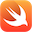```

and like this in K:

```f:{:[x!2;(4*x)-3;1+3*x]}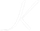```
Exercise: Which of these languages required that variables or functions be declared with types and which did not?
Exercise: Implement this function in PHP, Objective C, Ceylon, D, and Mercury.

## System Languages

System programming languages differ from application programming languages in that they are more concerned with managing a computer system rather than solving general problems in health care, game playing, or finance. In a system langauge, the programmer, not the runtime system, is generally responsible for:

• Memory management
• Process management
• Data transfer
• Caches
• Device drivers
• Directly interfacing with the operating system

## Scripting Languages

Scripting languages are used for wiring together systems and applications at a very high level. They are almost always extremely expressive (they do a lot with very little code) and usually dynamic (meaning the compiler does very little, while the run-time system does almost everything).

## Esoteric Languages

An esoteric language is one not intended to be taken seriously. They can be jokes, near-minimalistic, or despotic (purposely obfuscated or non-deterministic).

Exercise: Implement the function above in False, Brainfuck, Befunge, Malbolge, Kipple, and reMorse.

## Ousterhout’s Dichotomy

John Ousterhout once claimed that programming languages roughly fall into two types, which he called scripting and system languages. You can read about this idea at Wikipedia. Then read this two-part article (Part 1, Part 2) on the dichotomy and on languages that seem to reject it.